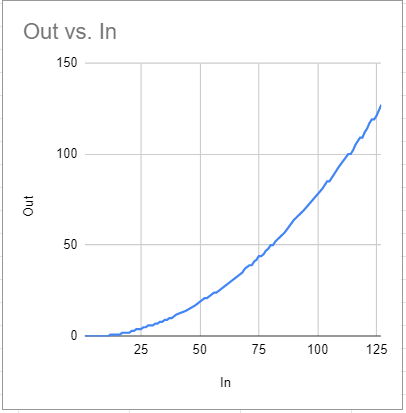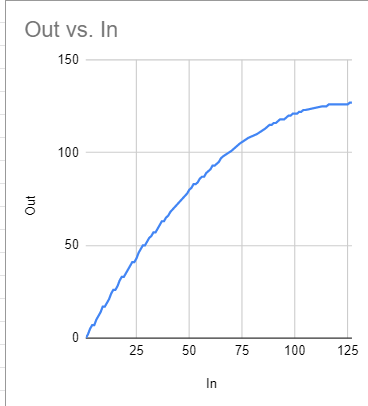# How to set rules to change cc value "0 to 127" onto "90 to 110"

How to set rules to change cc value “0 to 127” onto “90 to 110”
THX !!!

My thinking:

Input range 0-127 means 128 “units”.
Output range 90-110 means 20 “units”.
InRange/OutRange (in this case 128/20) = “MyUnit”
We can temporarily park this MyUnit in a variable rr for later use.

Now, if I divide the incoming value by MyUnit, I know what the current value is worth within the desired range. It is just 90 (minimum out range) too low, so I add 90.

I’m not good at math and I don’t know why this calculation is 90-111, but since it’s rough anyway I just limited the output.

My Example with incoming Channel 1, CC 28, value 0-127:

Input
Control Change
Channel 1 and set to ‘oo’
CC# 28 and set to ‘pp’
Value is “any value” and set to ‘qq’
swallow

Rules
rr = 128/20
qq = qq/rr
qq = qq+90
if qq>110 then qq=110

Output
Control Change
Channel ‘oo’
CC ‘pp’
Value ‘qq’

xx=the cc value between 0 and 127

xx=xx+1

tt=(xx/20)*128

ss=90+tt

ss=the cc value between 90 and 110

Thanks a lot my MIDI friends !!!

How is that supposed to work?
If I understand MT right, your formula must be written as:
xx=xx+1
tt=xx/20
tt=tt*128
ss=90+tt

But ‘tt’ goes up to 768 and ‘ss’ output stays stable at 90, perhaps because the high tt gets ignored.

``````76002: MIDI IN [Faderfox EC4]: B0 1C 7F
76003: IN   2.0  Control Change on ch. 1 set 'oo' to ch. with CC#:28 (0x1C) and 'pp' to CC# with any value and 'xx' to value=127
76004: RULE 2.0:1 expression: (xx=xx+1) = 128
76005: RULE 2.0:2 expression: (tt=xx/20) = 6
76006: RULE 2.0:3 expression: (tt=tt*128) = 768
76007: RULE 2.0:4 expression: (ss=90+tt) = 858
76008: MIDI OUT [Bome MIDI Translator 1 Virtual Out]: B0 1C 5A
``````

When I disable the ‘tt*128’ line, output is 90-96.
Did I something wrong or does it not work this way?

I am asking because range scaling will be quite common in my future setup and I want to learn the best techniques.

Here is a tutorial on scaling.

Keep in mind since Bome MIDI Translator does integer math and that the values are limited to 128 steps ( for CCs) that rounding errors may occur an in such a case, you might need to add a few tweaks if you are not getting the value you want.

Also keep in mind to do multiplication before division.

Steve

Ah, straight forward, nice!
Thank you.

Do you have any clever idea to add curve functions for such scaling other than mapping single values?

Velocity curves in MT Pro are a bit of a challenge as more advanceg math may be required especially when working with curves, integer arithmetic and limited values. With that said, the attached example might help. The key here is to define the appropriate mid value, control point and deviation value. Translator 0.1 is the one to look at the rules, define (in comments) the formula used and then in MT Pro available rules, how to apply them.

Again, not for the faint of heart but at least this is something that might help.

I actually plotted out two curves using this tool into a spreadsheet and the graphed it.Steve Caldwell
Bome Customer Care

Also available for paid consulting services: bome@sniz.biz

A very clever solution indeed. Not that I understand everything right away, it gives me a lot to think about. But the curves are definitely good enough to, for example, control two parameters and/or instruments with variation from the same source.

Thanks very much!

The right operation is to divide the cc by 128 and multiply it by 20:

xx=the cc value between 0 and 127
xx=xx+1
tt=(xx/128)
tt=tt*20
ss=90+tt
ss=the cc value between 90 and 110

example for maximum value:
xx=127
xx+1=128
tt=128/128=1
tt=1*20=20
ss=90+20=110
ss=110

example for minimum value:
xx=0
xx+1=1
tt=1/128=0,007=0
tt=0*20=0
ss=90+0=90
ss=90

example for mid value:
xx=64
xx+1=65
tt=65/128=0,5078125=0,50
tt=0,5*20=10
ss=90+10=100
ss=100

etc.

@jordikt

You should always do the multiplication first then the division since we are using integer arithmetic.

Steve Caldwell
Bome Customer Care

Also available for paid consulting services: bome@sniz.biz

@SteveC
Do you mean that dividing 4 by 1 inside bome results 0 instead of 0,25?

@jordikt

Yes